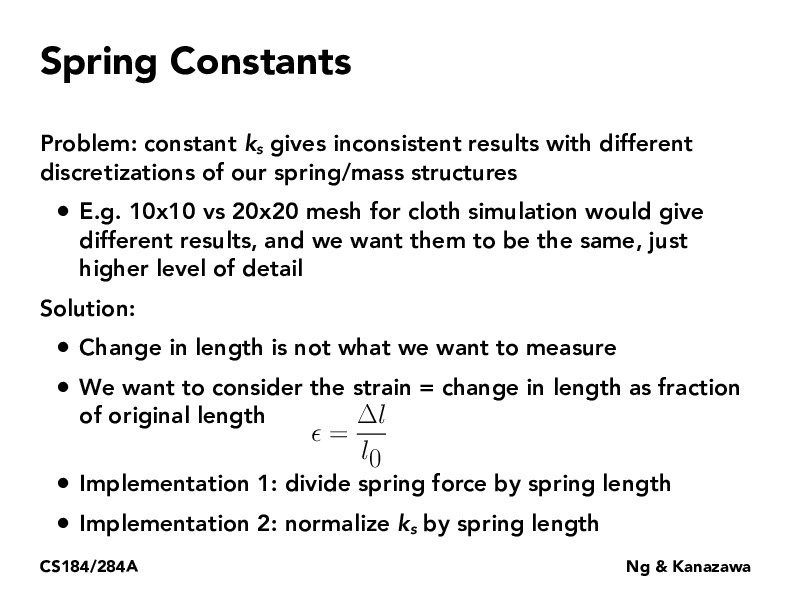Lecture 18: Introduction to Physical Simulation (17)DanielMolina24

I don't quite understand why we would want to consider strain. I thought to recompute the new stiffness coefficient we could just calculate F/(new displacement) since F = ks * displacementdaniswords

I don't have a thorough answer to the question above, but this is how I made sense of it in my mind: imagine we had 2 different length springs, one much larger than the other. Now imagine that there is a length change in both that is the same amount. This same amount is a much larger proportion of the smaller spring. So it seems to me that just looking at change in length doesn't give us enough information.sayandas00

Is the spring length on this slide for normalizing k_s in terms of mesh dimensions or in terms of some length in the scene? Because it seems like the example of the 10x10 vs 20x20 mesh implies both meshes take up the same amount of length in the scene, so I wasn't sure which quantity to use.

You must be enrolled in the course to comment